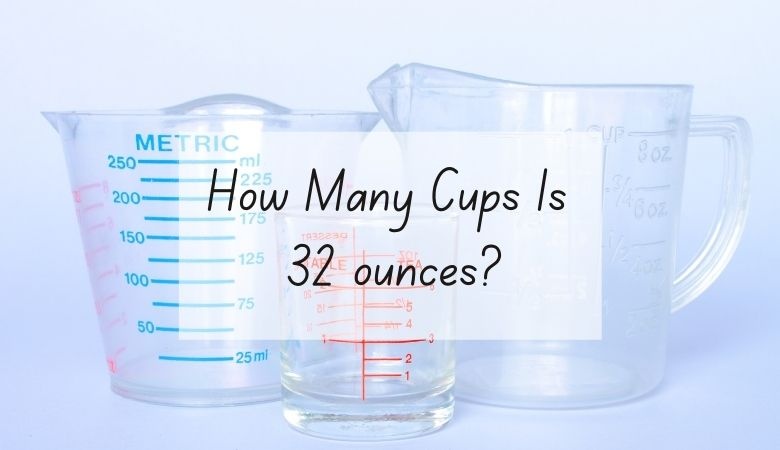# How Many Cups Is 32 oz?

32 ounces is equal to 4 cups.

Although cups and ounces are both units of measurement in the same system, converting between the two can sometimes be a bit challenging.

However, both 1 cup and 32 ounces are common measurements, so getting a feel for the two and learning to convert between them can be very helpful.

One US ounce equals .125 cups. This means that, in order to figure out how many cups are in a certain number of ounces, you need to divide the number of ounces by eight.

For example, when calculating how many cups are in 32 ounces, you would divide 32 by eight.

The answer, which is four, tells you that there are 4 cups in 32 ounces.

You can also reverse the equation to figure out how many ounces there are in any given number of cups. For this, you would simply need to multiply the number of cups by eight.

For example, if you have 6 cups, you would multiply 6 by 8. The answer, which is 48, tells you that there are 48 ounces in 6 cups.

This equation also tells us that there are 8 ounces in a single cup.

If you don’t want to do the math, this conversion calculator makes it easy to convert ounces into cups.

Here is an ounces into cups conversion table for you to reference.

## Cups and ounces units of measurement

Cups and ounces are common measurements used in the United States, particularly for cooking or baking.

While cups are used to measure both wet and dry ingredients, ounces are most often used to measure fluids.

Most measuring vessels have both ounces and cups printed on them, which makes it easy to accurately measure or convert different food items.